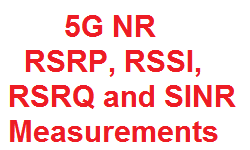# 5G NR Measurements: RSRP, RSSI, RSRQ and SINR

In LTE, the UE measurements are done for RSRP, RSRQ and SNR associated with CRS (Cell Specific Reference Signal) .  5G NR uses  SS (Synchronization Signal) and CSI (Channel State Information) instead of CRS, so 3GPP had put new definitions for UE measurements in place, and some of these are discussed below.

• For FR-1, the reference point for the measurement shall be the antenna connector of the UE.
• For FR-2, it shall be measured based on the combined signal from antenna elements corresponding to a given receiver branch.

SS-RSRP: SS-RSRP stands for Synchronization Signal reference signal received power. It is defined as the linear average over the power contributions (in Watt) of the resource elements that carry SSS. The measurement time resource(s) for SS-RSRP are confined within SS/PBCH Block Measurement Time Configuration (SMTC) window duration.

For SS-RSRP determination, demodulation reference signals for PBCH and, if indicated by higher layers, CSI RS in addition to SSS may be used.. SS-RSRP using demodulation reference signal for PBCH or CSI reference signal shall be measured by linear averaging over the power contributions of the resource elements that carry corresponding reference signals taking into account power scaling for the reference signals. This measurement is applicable for following:

• RRC_CONNECTED intra-frequency
• RRC_IDLE intra-frequency
• RRC_IDLE inter-frequency
• RRC_INACTIVE intra-frequency
• RRC_INACTIVE inter-frequency
• RRC_CONNECTED intra-frequency
• RRC_CONNECTED inter-frequency

CSI-RSRP: CSI-RSRP stands for CSI reference signal received power. It is defined as the linear average over the power contributions (in watt) of the resource elements that carry CSI-RS configured for RSRP measurements within the considered measurement frequency bandwidth in the configured CSI-RS occasions. For CSI-RSRP determination CSI reference signals transmitted on antenna port 3000 shall be used, if CSI_RSRP is used for L1-RSRP, CSI reference signals transmitted on antenna ports 3000, 3001 can be used.

For intra-frequency CSI-RSRP measurements, if the measurement gap is not configured, UE is not expected to measure the CSI-RS resource(s) outside of the active downlink bandwidth part. CSI-RSRP measurement is applicable for following:

• If CSI-RSRP is used for L1-RSRP, RRC_CONNECTED intra-frequency.
Otherwise,
• RRC_CONNECTED intra-frequency,
• RRC_CONNECTED inter-frequency

NR-RSSI: NR-RSSI  stands for NR carrier Received Signal Strength Indicator, it comprises the linear average of the total received power (in Watt) observed only in certain OFDM symbols of measurement time resource(s), in the measurement bandwidth, over N number of resource blocks from all sources, including co-channel serving and non-serving cells, adjacent channel interference, thermal noise etc. The measurement time resource(s) for NR Carrier RSSI are confined within SS/PBCH Block Measurement Time Configuration (SMTC) window duration.

• For intra-frequency measurements, NR Carrier RSSI is measured with timing reference corresponding to the serving cell in the frequency layer
• For inter-frequency measurements, NR Carrier RSSI is measured with timing reference corresponding to any cell in the target frequency layer

CSI-RSSI: CSI-RSSI stands for CSI Received Signal Strength Indicator, it comprises the linear average of the total received power (in Watt) observed only in OFDM symbols of measurement time resource(s), in the measurement bandwidth, over N number of resource blocks from all sources, including cochannel serving and non-serving cells, adjacent channel interference, thermal noise etc. The measurement time resource(s) for CSI-RSSI corresponds to OFDM symbols containing configured CSI-RS occasions.

SS-RSRQ: SS-RSRQ stands for Secondary synchronization Signal Reference Signal Received Quality. It is defined as the ratio of N×SS-RSRP /NR carrier RSSI, where N is the number of resource blocks in the NR carrier RSSI measurement bandwidth. The measurements in the numerator and denominator shall be made over the same set of resource blocks. SS-RSRQ measurement is applicable for following:

• RRC_IDLE intra-frequency
• RRC_IDLE inter-frequency
• RRC_INACTIVE intra-frequency
• RRC_INACTIVE inter-frequency
• RRC_CONNECTED intra-frequency
• RRC_CONNECTED inter-frequency

CSI-RSRQ: is defined as the ratio of N×CSI-RSRP to CSI-RSSI, where N is the number of resource blocks in the RSSI measurement bandwidth. The measurements in the numerator and denominator shall be made over the same set of resource blocks. CSI-RSRQ measurement is applicable for following:

• RRC_CONNECTED intra-frequency
• RRC_CONNECTED inter-frequency

SS-SINR: SS-SINR stands for SS signal-to-noise and interference ratio. It is defined as the linear average over the power contribution (in Watt) of the resource elements carrying SSS divided by the linear average of the noise and interference power contribution (in Watt) over the resource elements carrying SSS within the same frequency bandwidth. SS-SINR measurement is applicable for following:

• RRC_CONNECTED intra-frequency
• RRC_CONNECTED inter-frequency

CSI-SINR: CSI-SINR stands for CSI signal-to-noise and interference ratio.It is defined as the linear average over the power contribution (in Watt) of the resource elements carrying CSI reference signals divided by the linear average of the noise and interference power contribution (in Watt) over the resource elements carrying CSI reference signals within the same frequency bandwidth.SS-SINR measurement is applicable for following:

• RRC_CONNECTED intra-frequency
• RRC_CONNECTED inter-frequency

References:

• 3GPP TS 38.215: 5G NR; Physical layer measurements
• 3GPP TS 38.213:5G NR; Physical layer procedures for control channels
• 3GPP TS 38.214: 5G NR; Physical layer procedures for data channels

Related Posts: